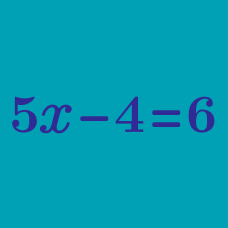Algebra

# Simple Equations

What value of $$x$$ satisfies the following equation: $$x + 5 = 18$$?

What is the value of $$x$$ that satisfies the equation $x+8=40?$

What is the value of $$x$$ that satisfies the equation $$6x = 18$$?

If $$4x + 11 = 67$$, what is the value of $$x$$?

What is the value of $$x$$ that satisfies the equation $$\dfrac{x}{3} = 9$$?

×

Problem Loading...

Note Loading...

Set Loading...